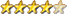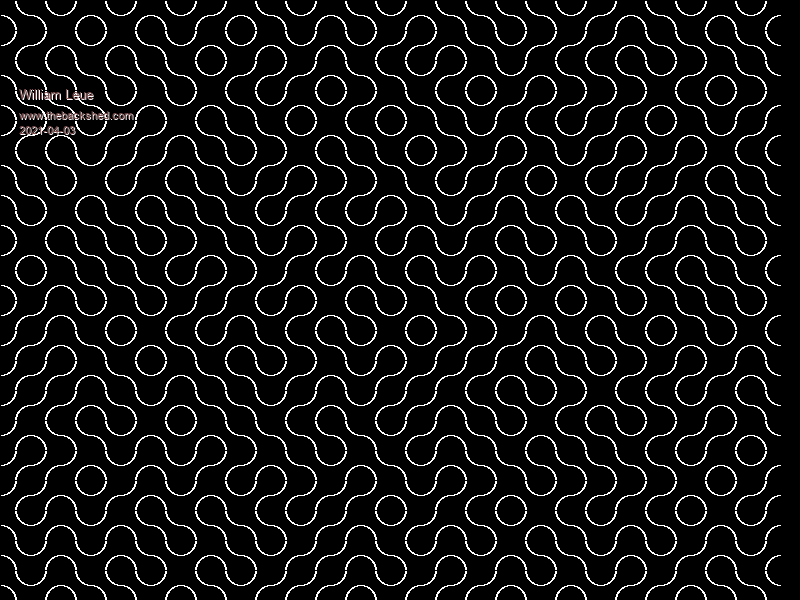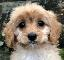Simple Maze using Truchet Tiles

Author Message
William Leue
GuruJoined: 03/07/2020
Location: United States
Posts: 331
 Posted: 03:14pm 02 Apr 2021

I'm tired of wrestling with the code for some of my more ambitious CMM2 projects, so here's a tiny little program that creates a sort-of maze using Truchet Tiles (see Wikipedia). It has a very close relationship to the famous one-line Commodore Pet maze generator known as '10Print'.

' Maze formed from Truchet Tiles
' This is basically the same algorithm as the famous
' Commodore Pet '10Print' one-line maze.
' Rev 1.0.0 William M Leue 4/1/2021
option default integer
option base 1

const TSIZE = 30

cls
ncols = MM.HRES\TSIZE
nrows = MM.VRES\TSIZE
for row = 1 to nrows
y = (row-1)*TSIZE
for col = 1 to ncols
x = (col-1)*TSIZE
DrawTruchetTile x, y, rnd()
next col
next row
end

sub DrawTruchetTile x, y, r as float
local cx, cy
if r < 0.5 then
cx = x : cy = y + TSIZE
arc cx, cy, TSIZE\2,, 0, 90
cx = x + TSIZE : cy = y
arc cx, cy, TSIZE\2,, 180, 270
else
cx = x: cy = y
arc cx, cy, TSIZE\2,, 90, 180
cx = x + TSIZE: cy = y + TSIZE
arc cx, cy, TSIZE\2,, -90, 0
end if
end subvegipeteGuruJoined: 29/01/2013
Posts: 971
 Posted: 12:01am 03 Apr 2021

Cool!

Here's a slight variation. I fudged the numbers slightly to get joined arcs, extended the loops to go past the screen edges, put a box around the perimeter and added a flood fill to colour a portion of the 'maze'. The red dot shows the start of the flood fill. Press any key for another image, [Esc] to quit.

Edit: added the option for corners instead of curves. Press "1" to change to corners, "0" to change to curves.

It would be handy to know how many pixels the flood fill command actually drew...

' Maze formed from Truchet Tiles
' This is basically the same algorithm as the famous
' Commodore Pet '10Print' one-line maze.
' Rev 1.0.0 William M Leue 4/1/2021
option default integer
option base 1

const TSIZE = 30
CORNERS = 0

do
cls
ncols = MM.HRES\TSIZE
nrows = MM.VRES\TSIZE
for row = 1 to nrows+1
y = (row-1)*TSIZE
for col = 1 to ncols+1
x = (col-1)*TSIZE
DrawTruchetTile x, y, rnd()
next col
next row
box 0,0,MM.HRES,MM.VRES
pixel fill ncols*TSIZE/2,nrows*TSIZE/2,rgb(blue)
circle ncols*TSIZE/2,nrows*TSIZE/2,TSIZE/4,0,1,,rgb(red)
do : k\$ = inkey\$ : loop until k\$ <> ""
if k\$ = "1" then CORNERS = 1
if k\$ = "0" then CORNERS = 0
loop until k\$ = chr\$(27)
end

sub DrawTruchetTile x, y, r as float
if CORNERS then
if r < 0.5 then
line x + TSIZE/2, y, x + TSIZE, y + TSIZE/2
line x, y + TSIZE/2, x + TSIZE/2, y + TSIZE
else
line x + TSIZE/2, y, x, y + TSIZE/2
line x + TSIZE, y + TSIZE/2, x + TSIZE/2, y + TSIZE
end if
else
if r < 0.5 then
arc x, y + TSIZE, TSIZE/2,, 0, 93
arc x + TSIZE, y, TSIZE/2,, 180, 273
else
arc x, y, TSIZE/2,, 87, 180
arc x + TSIZE, y + TSIZE, TSIZE/2,, -93, 0
end if
end if
end sub

Edited 2021-04-03 10:20 by vegipete

TassyJimGuruJoined: 07/08/2011
Location: Australia
Posts: 5424
 Posted: 02:14am 03 Apr 2021

Very nice.
To go one step further, I added mouse control

Click anywhere to show the connected paths

' Maze formed from Truchet Tiles
' This is basically the same algorithm as the famous
' Commodore Pet '10Print' one-line maze.
' Rev 1.0.0 William M Leue 4/1/2021
OPTION DEFAULT INTEGER
OPTION BASE 1
DIM INTEGER oldX, newX, oldY, newY
CONST TSIZE = 30
CORNERS = 0
mouse_port = MM.INFO(OPTION MOUSE)
IF mouse_port <> -1 THEN ' we have a mouse
CONTROLLER MOUSE OPEN mouse_port
GUI CURSOR ON 0,MOUSE(x,mouse_port), MOUSE(y,mouse_port)
ENDIF
DO
CLS
ncols = MM.HRES\TSIZE
nrows = MM.VRES\TSIZE
FOR row = 1 TO nrows+1
y = (row-1)*TSIZE
FOR col = 1 TO ncols+1
x = (col-1)*TSIZE
DrawTruchetTile x, y, RND()
NEXT col
NEXT row
BOX 0,0,MM.HRES,MM.VRES

DO : k\$ = INKEY\$
GUI CURSOR MOUSE(X, mouse_port), MOUSE(Y, mouse_port)
IF MOUSE(L,mouse_port) THEN mouseclick
LOOP UNTIL k\$ <> ""
IF k\$ = "1" THEN CORNERS = 1
IF k\$ = "0" THEN CORNERS = 0
LOOP UNTIL k\$ = CHR\$(27)

IF mouse_port <> -1 THEN ' we have a mouse
CONTROLLER MOUSE CLOSE mouse_port
GUI CURSOR OFF
ENDIF
END

SUB DrawTruchetTile x, y, r AS FLOAT
IF CORNERS THEN
IF r < 0.5 THEN
LINE x + TSIZE/2, y, x + TSIZE, y + TSIZE/2
LINE x, y + TSIZE/2, x + TSIZE/2, y + TSIZE
ELSE
LINE x + TSIZE/2, y, x, y + TSIZE/2
LINE x + TSIZE, y + TSIZE/2, x + TSIZE/2, y + TSIZE
END IF
ELSE
IF r < 0.5 THEN
ARC x, y + TSIZE, TSIZE/2,, 0, 93
ARC x + TSIZE, y, TSIZE/2,, 180, 273
ELSE
ARC x, y, TSIZE/2,, 87, 180
ARC x + TSIZE, y + TSIZE, TSIZE/2,, -93, 0
END IF
END IF
END SUB

SUB mouseClick
newX = INT((MOUSE(X, mouse_port)+tsize/2)/tsize)*tsize
newY = INT((MOUSE(Y, mouse_port)+tsize/2)/tsize)*tsize
GUI CURSOR HIDE
IF oldX <>0 THEN
PIXEL FILL oldX,oldY,RGB(BLUE) ' hide the red dot
PIXEL FILL oldX,oldY,RGB(BLACK)
ENDIF
PIXEL FILL newX,newY,RGB(BLUE)
CIRCLE newX,newY,TSIZE/4,0,1,,RGB(RED)
oldX = newX
oldY = newY
GUI CURSOR SHOW
END SUB

I have assumed that you have a mouse set with OPTION MOUSE n

Jim

William Leue
GuruJoined: 03/07/2020
Location: United States
Posts: 331
 Posted: 08:20pm 05 Apr 2021

I also some bling to mine: the screen now scrolls continuously upward as new rows are added at the bottom, and the color of the swirls now varies slowly and continuously around the wheel of fully saturated bright colors.

-Bill

' Maze formed from Truchet Tiles
' This is basically the same algorithm as the famous
' Commodore Pet '10Print' one-line maze.
' Rev 1.0.0 William M Leue 4/1/2021
option base 1

const TSIZE = 30

mode 1,16
open "debug.txt" for output as #1
cls
ncols = MM.HRES\TSIZE
nrows = MM.VRES\TSIZE
dim float h = 0.0
dim float s = 1.0
dim float v = 1.0
for row = 1 to nrows-1
y = (row-1)*TSIZE
for col = 1 to ncols
x = (col-1)*TSIZE
HSV2RGB(h, s, v, r, g, b)
c = RGB(r, g, b)
DrawTruchetTile x, y, rnd(), c
next col
next row
do
page scroll 0, 0, TSIZE, -1
y = (nrows-2)*TSIZE
for col = 1 to ncols
x = (col-1)*TSIZE
HSV2RGB(h, s, v, r, g, b)
c = RGB(r, g, b)
DrawTruchetTile x, y, rnd(), c
next col
pause 200
h = h + 0.5
if h > 360.0 then h = 0.0
print #1, "HSV2RGB(" + str\$(h) + "," + str\$(s) + "," + str\$(v) + "," + str\$(r) + "," + str\$(g) + "," +  str\$(b)
loop
end

sub DrawTruchetTile x, y, r as float, c
local cx, cy
if r < 0.5 then
cx = x : cy = y + TSIZE
arc cx, cy, TSIZE\2,, 0, 90, c
cx = x + TSIZE : cy = y
arc cx, cy, TSIZE\2,, 180, 270, c
else
cx = x: cy = y
arc cx, cy, TSIZE\2,, 90, 180, c
cx = x + TSIZE: cy = y + TSIZE
arc cx, cy, TSIZE\2,, -90, 0, c
end if
end sub

' ColorConvert.inc -- BASIC include file suitable for inclusion
' wherever color specs using RGB or HSV need to be inter-converted.
' William M Leue 1/7/2021

' Convert an RGB Color to HSV values.
' the RGB values must be in range 0..255; the S and V values will
' be in range 0..1; the H value will be in range 0..360
sub RGB2HSV r, g, b, h as float, s as float, v as float
local float rp, cmax, cmin, delta

rp = r/255.0 : gp = g/255.0 : bp = b/255.0
cmax = max(rp, max(gp, bp))
cmin = min(rp, min(gp, bp))
delta = cmax - cmin
if delta = 0 then
h = 0
else if cmax = rp then
h = 60*(((gp-bp)/delta) MOD 6)
else if cmax = gp then
h = 60*(((bp-rp)/delta) + 2)
else
h = 60*(((rp-gp)/delta) + 4)
end if
if cmax = 0 then
s = 0
else
s = delta/cmax
end if
v = cmax
end sub

' Convert an HSV value to its RGB equivalent
' The S and V values must be in range 0..1; the H value must
' be in range 0..360. The RGB values will be in range 0..255.
sub HSV2RGB h as float, s as float, v as float, r, g, b
local float hh, f, p, q, t, x, c, i
'local i
c = v*s
hh = h/60.0
i = int(hh)
f = hh - i
p = v*(1-s)
q = v*(1-s*f)
t = v*(1-s*(1-f))
x = c*(1.0 - hi MOD 2 - 1)

select case i
case 0
rp = v : gp = t : bp = p
case 1
rp = q : gp = v : bp = p
case 2
rp = p : gp = v : bp = t
case 3
rp = p : gp = q : bp = v
case 4
rp = t : gp = p : bp = v
case 5
rp = v : gp = p : bp = q
end select
r = rp*255 : g = gp*255 : b = bp*255
end sub

' function to return an RGB color, given the h, s, and v
' values as input parameters. The S and V values must be
' in the range 0..1; the H value must be in the range
' 0..360. The output value will be a 24-bit RGB color.
function GetRGBColor(h as float, s as float, v as float)
local r, g, b, c

HSV2RGB h, s, v, r, g, b
c = RGB(r ,g, b)
GetRGBColor = c
end function

' draw color swatches: the left-hand one is the original
' RGB color specified by the user. The right-hand one is
' that color first converted from RGB to HSV, and then
' back again to RGB. The two swatches will be the
' identical color.
sub ShowColors r,g,b,r2,g2,b2
local c1, c2

c1 = RGB(r,g,b)
c2 = RGB(r2,g2,b2)
box 200,200,100,100,1, RGB(WHITE), c1
box 400,200,100,100,1, RGB(WHITE), c2
end sub

sub ShowPixelColors r,g,b
local c1, x, y

box 200,400,100,100,1, RGB(WHITE), c1
c1 = RGB(r,g,b)
for y = 401 to 498
for x = 201 to 298
pixel x, y, c1
next x
next y
end sub

William Leue
GuruJoined: 03/07/2020
Location: United States
Posts: 331
 Posted: 08:21pm 05 Apr 2021

Oops, you probably will want to remove the 'print #1' statement so as not to fill up your SD card with debugging crap.

-Bill